# How to Combine Models with Stacking

Administrator, Moderator, Employee, RapidMiner Certified Analyst, RapidMiner Certified Expert, University Professor Posts: 2,504RM Data Scientist
edited November 2018

At some point in your analysis you come at the point where you want to boost your model performance. The first step for this would be to go in the feature generation phase and search for better attribute combination for your learners.

As a next step, you might want to boost the performance of your machine learning method. A pretty common approach for this is called ensemble learning. In ensemble learning you build a lot of different (base) learners. The results of these base learners are combined or chained in different ways. In this article, we will focus on a technique called Stacking. Other approaches are Voting, Bagging or Boosting. These methods also available in RapidMiner.

In Stacking you have at least two algorithms called base learners. These learners do what they do if you would train them on the data set itself separately. You use the base learners on the data set which results in adata set containing your usual attributes and the prediction of your base learners.

Afterwards you use another algorithm on this enriched data set which uses the original attributes and the results of the previous learning step.

In essence, you use two algorithms to build an enriched data set so that a third algorithm can deliver better results.

## Problem and Learners

To illustrate this problem, we have a look at a problem called Checkerboard. The data has two attributes att1 and att2 and is structured with square patches belonging to one group.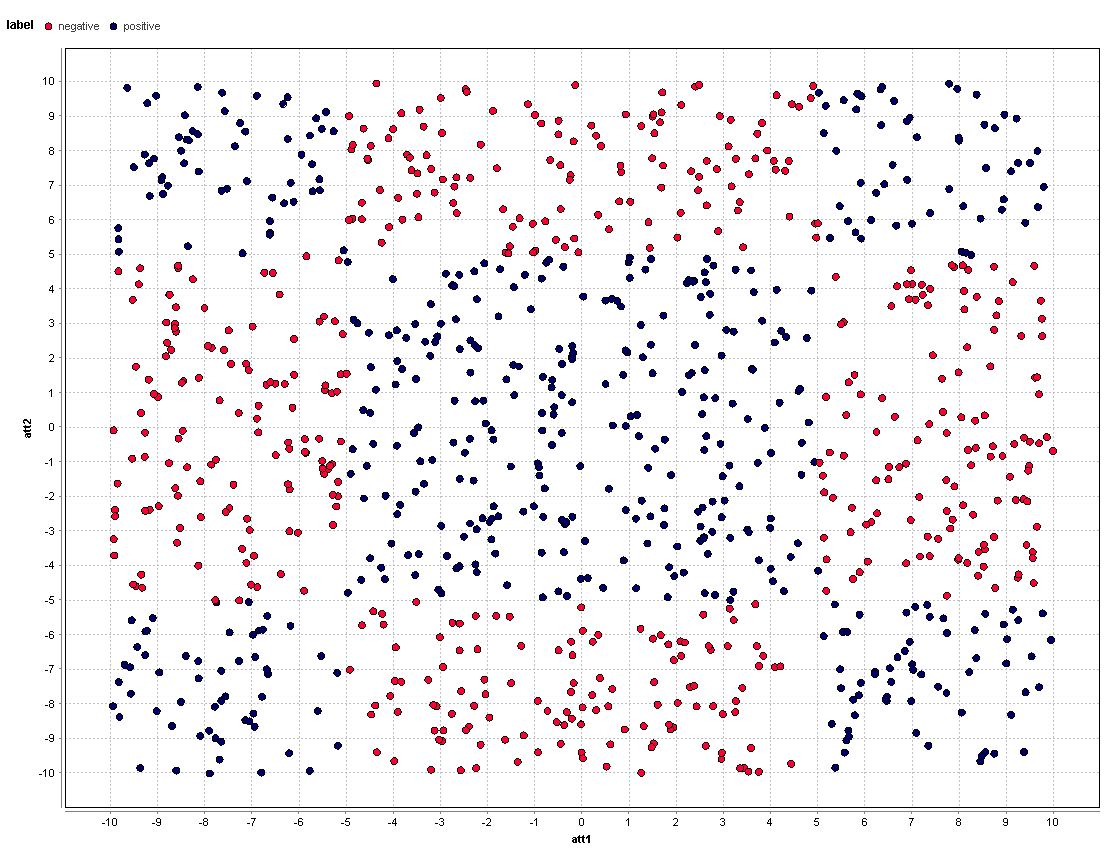Let’s try to solve this problem with a few learners and see what they can archive. To see what the algorithm found we can apply our model on random data. Afterwards we do a scatter plot with prediction on the colour axis to investigate decision boundaries. The results for each algorithm are depicted below.

Naïve Bayes: By design Naïve Bayes can only model a n-dimensional ellipsoid. Because we are working in two dimensions, Naïve Bayes tries to find the most discriminating ellipse. As seen below it puts one on the origin. This is the only pattern it can recognize.

k-NN: We also try k-NN with cosine similarity as distance measure (Euclidian distance would solve the problem well on its own). With cosine similarity k-NN can find angular regions as a pattern. The result is that k-NN finds a star like pattern and recognizes that the corners are blue. It fails to recognize the central region as a blue area.

Decision Tree: A Decision Tree model fails to discriminate. The reason for this is, that the decision tree looks at each dimension separately. But in each dimension the data is uniformly distributed. A Decision Tree finds no cut to solve this.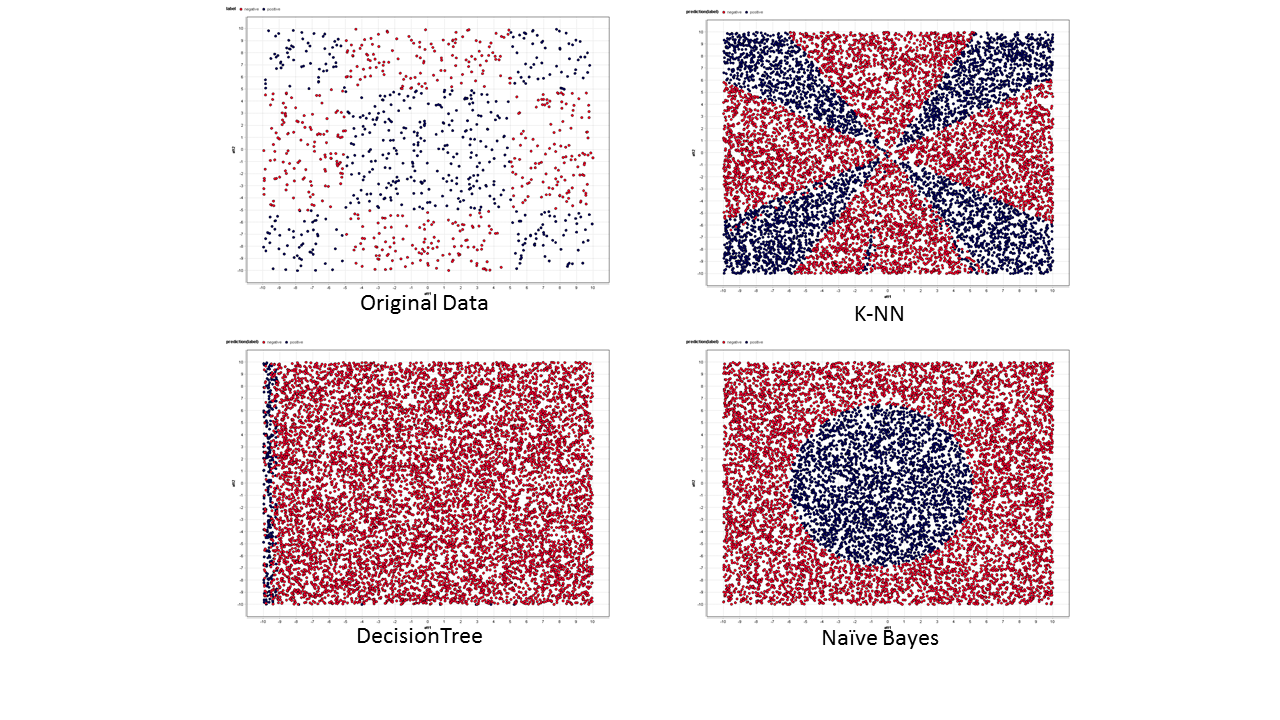## Stacking

Now, let’s “stack” these algorithms together. We use k-NN and Naïve Bayes as a base learner and Decision Tree to combine the results.

The decision tree will get the results of both base learners as well as the original attributes as input: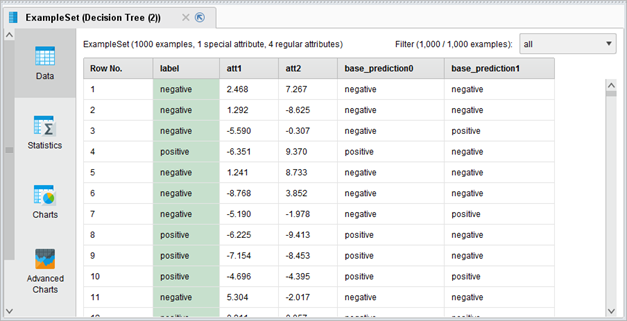Where base_prediction0 is the result of Naïve Bayes and base_prediction1 is the result of k-NN. The tree can thus pick regions where it trusts different algorithms. In those areas, the tree can even split into smaller regions. The resulting tree looks like this: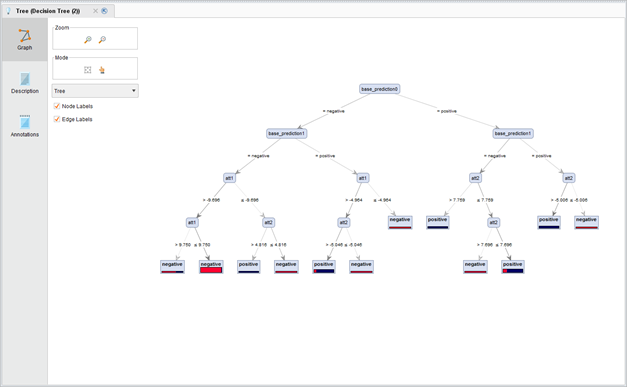Applied on random test data we get a result which is depicted below.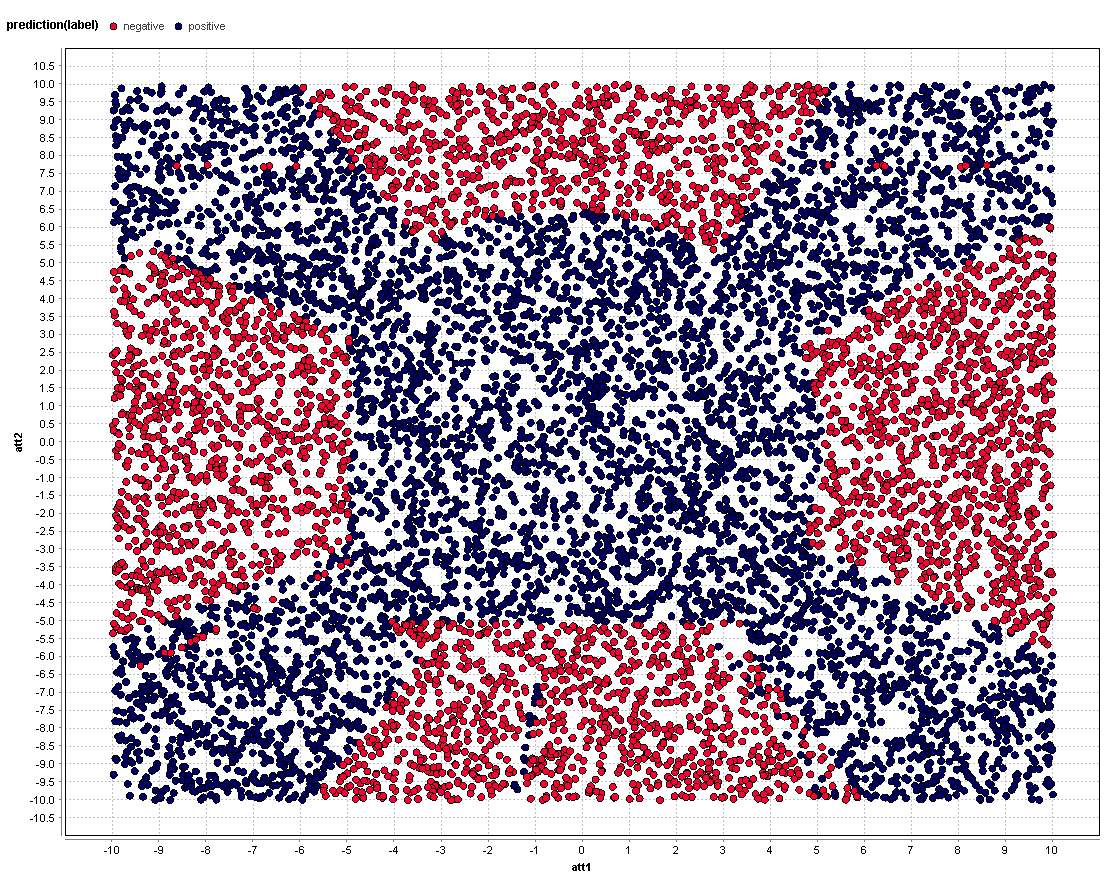This is an impressive result. We take two learners which are not creating good results by their own and combine them with a learner which was not able to do anything on the data set and get a good result.

- Head of Data Science Services at RapidMiner -
Dortmund, Germany

• Member Posts: 3Contributor I

Hi! Thank you for the nice article. Please, could you post the xml file or a link with the process and dataset.

Thank you.

• Member Posts: 1Contributor I

Hi @mschmitz,

First, thanks for all the great work and nice tutorial on stacking. Is it possible to create a stacking of other stackings? Sort of like a meta-meta-model. I know this has been done in data competitions (i.e., Kaggle) and I was just testing it out. Below there's an xml of a process I created using the 'stacking' tutorial process in RM as the basis. I'm getting the following message:

I also tried a variant of this which was use a vote operator on multiple stacking operators but unfortunately I got the same 'process failed' message.

`<?xml version="1.0" encoding="UTF-8"?><process version="7.3.001">  <context>    <input/>    <output/>    <macros/>  </context>  <operator activated="true" class="process" compatibility="6.0.002" expanded="true" name="Root">    <parameter key="logverbosity" value="init"/>    <parameter key="random_seed" value="2001"/>    <parameter key="send_mail" value="never"/>    <parameter key="notification_email" value=""/>    <parameter key="process_duration_for_mail" value="30"/>    <parameter key="encoding" value="SYSTEM"/>    <process expanded="true">      <operator activated="true" class="retrieve" compatibility="7.3.001" expanded="true" height="68" name="Sonar" width="90" x="313" y="34">        <parameter key="repository_entry" value="//Samples/data/Sonar"/>      </operator>      <operator activated="true" class="split_validation" compatibility="7.3.001" expanded="true" height="124" name="Validation" width="90" x="514" y="34">        <parameter key="create_complete_model" value="false"/>        <parameter key="split" value="relative"/>        <parameter key="split_ratio" value="0.7"/>        <parameter key="training_set_size" value="100"/>        <parameter key="test_set_size" value="-1"/>        <parameter key="sampling_type" value="shuffled sampling"/>        <parameter key="use_local_random_seed" value="false"/>        <parameter key="local_random_seed" value="1992"/>        <process expanded="true">          <operator activated="true" class="stacking" compatibility="7.3.001" expanded="true" height="68" name="Stacking" width="90" x="112" y="30">            <parameter key="keep_all_attributes" value="true"/>            <process expanded="true">              <operator activated="true" class="stacking" compatibility="7.3.001" expanded="true" height="68" name="Stacking (2)" width="90" x="179" y="34">                <parameter key="keep_all_attributes" value="true"/>                <process expanded="true">                  <operator activated="true" class="parallel_decision_tree" compatibility="7.3.001" expanded="true" height="82" name="Decision Tree" width="90" x="112" y="34">                    <parameter key="criterion" value="gain_ratio"/>                    <parameter key="maximal_depth" value="20"/>                    <parameter key="apply_pruning" value="true"/>                    <parameter key="confidence" value="0.25"/>                    <parameter key="apply_prepruning" value="true"/>                    <parameter key="minimal_gain" value="0.1"/>                    <parameter key="minimal_leaf_size" value="2"/>                    <parameter key="minimal_size_for_split" value="4"/>                    <parameter key="number_of_prepruning_alternatives" value="3"/>                  </operator>                  <operator activated="true" class="k_nn" compatibility="7.3.001" expanded="true" height="82" name="K-NN" width="90" x="112" y="136">                    <parameter key="k" value="5"/>                    <parameter key="weighted_vote" value="false"/>                    <parameter key="measure_types" value="MixedMeasures"/>                    <parameter key="mixed_measure" value="MixedEuclideanDistance"/>                    <parameter key="nominal_measure" value="NominalDistance"/>                    <parameter key="numerical_measure" value="EuclideanDistance"/>                    <parameter key="divergence" value="GeneralizedIDivergence"/>                    <parameter key="kernel_type" value="radial"/>                    <parameter key="kernel_gamma" value="1.0"/>                    <parameter key="kernel_sigma1" value="1.0"/>                    <parameter key="kernel_sigma2" value="0.0"/>                    <parameter key="kernel_sigma3" value="2.0"/>                    <parameter key="kernel_degree" value="3.0"/>                    <parameter key="kernel_shift" value="1.0"/>                    <parameter key="kernel_a" value="1.0"/>                    <parameter key="kernel_b" value="0.0"/>                  </operator>                  <operator activated="true" class="linear_regression" compatibility="7.3.001" expanded="true" height="103" name="Linear Regression" width="90" x="112" y="238">                    <parameter key="feature_selection" value="M5 prime"/>                    <parameter key="alpha" value="0.05"/>                    <parameter key="max_iterations" value="10"/>                    <parameter key="forward_alpha" value="0.05"/>                    <parameter key="backward_alpha" value="0.05"/>                    <parameter key="eliminate_colinear_features" value="true"/>                    <parameter key="min_tolerance" value="0.05"/>                    <parameter key="use_bias" value="true"/>                    <parameter key="ridge" value="1.0E-8"/>                  </operator>                  <connect from_port="training set 1" to_op="Decision Tree" to_port="training set"/>                  <connect from_port="training set 2" to_op="K-NN" to_port="training set"/>                  <connect from_port="training set 3" to_op="Linear Regression" to_port="training set"/>                  <connect from_op="Decision Tree" from_port="model" to_port="base model 1"/>                  <connect from_op="K-NN" from_port="model" to_port="base model 2"/>                  <connect from_op="Linear Regression" from_port="model" to_port="base model 3"/>                  <portSpacing port="source_training set 1" spacing="0"/>                  <portSpacing port="source_training set 2" spacing="0"/>                  <portSpacing port="source_training set 3" spacing="0"/>                  <portSpacing port="source_training set 4" spacing="0"/>                  <portSpacing port="sink_base model 1" spacing="0"/>                  <portSpacing port="sink_base model 2" spacing="0"/>                  <portSpacing port="sink_base model 3" spacing="0"/>                  <portSpacing port="sink_base model 4" spacing="0"/>                </process>                <process expanded="true">                  <operator activated="true" class="naive_bayes" compatibility="7.3.001" expanded="true" height="82" name="Naive Bayes (2)" width="90" x="123" y="30">                    <parameter key="laplace_correction" value="true"/>                  </operator>                  <connect from_port="stacking examples" to_op="Naive Bayes (2)" to_port="training set"/>                  <connect from_op="Naive Bayes (2)" from_port="model" to_port="stacking model"/>                  <portSpacing port="source_stacking examples" spacing="0"/>                  <portSpacing port="sink_stacking model" spacing="0"/>                </process>              </operator>              <operator activated="true" class="stacking" compatibility="7.3.001" expanded="true" height="68" name="Stacking (3)" width="90" x="179" y="136">                <parameter key="keep_all_attributes" value="true"/>                <process expanded="true">                  <operator activated="true" class="h2o:deep_learning" compatibility="7.3.000" expanded="true" height="82" name="Deep Learning" width="90" x="112" y="34">                    <parameter key="activation" value="Rectifier"/>                    <enumeration key="hidden_layer_sizes">                      <parameter key="hidden_layer_sizes" value="50"/>                      <parameter key="hidden_layer_sizes" value="50"/>                    </enumeration>                    <enumeration key="hidden_dropout_ratios"/>                    <parameter key="reproducible_(uses_1_thread)" value="false"/>                    <parameter key="use_local_random_seed" value="false"/>                    <parameter key="local_random_seed" value="1992"/>                    <parameter key="epochs" value="10.0"/>                    <parameter key="compute_variable_importances" value="false"/>                    <parameter key="train_samples_per_iteration" value="-2"/>                    <parameter key="adaptive_rate" value="true"/>                    <parameter key="epsilon" value="1.0E-8"/>                    <parameter key="rho" value="0.99"/>                    <parameter key="learning_rate" value="0.005"/>                    <parameter key="learning_rate_annealing" value="1.0E-6"/>                    <parameter key="learning_rate_decay" value="1.0"/>                    <parameter key="momentum_start" value="0.0"/>                    <parameter key="momentum_ramp" value="1000000.0"/>                    <parameter key="momentum_stable" value="0.0"/>                    <parameter key="nesterov_accelerated_gradient" value="true"/>                    <parameter key="standardize" value="true"/>                    <parameter key="L1" value="1.0E-5"/>                    <parameter key="L2" value="0.0"/>                    <parameter key="max_w2" value="10.0"/>                    <parameter key="loss_function" value="Automatic"/>                    <parameter key="distribution_function" value="AUTO"/>                    <parameter key="early_stopping" value="false"/>                    <parameter key="stopping_rounds" value="1"/>                    <parameter key="stopping_metric" value="AUTO"/>                    <parameter key="stopping_tolerance" value="0.001"/>                    <parameter key="missing_values_handling" value="MeanImputation"/>                    <parameter key="max_runtime_seconds" value="0"/>                    <list key="expert_parameters"/>                    <list key="expert_parameters_"/>                  </operator>                  <operator activated="true" class="h2o:gradient_boosted_trees" compatibility="7.3.000" expanded="true" height="103" name="Gradient Boosted Trees" width="90" x="112" y="136">                    <parameter key="number_of_trees" value="20"/>                    <parameter key="reproducible" value="false"/>                    <parameter key="maximum_number_of_threads" value="4"/>                    <parameter key="use_local_random_seed" value="false"/>                    <parameter key="local_random_seed" value="1992"/>                    <parameter key="maximal_depth" value="5"/>                    <parameter key="min_rows" value="10.0"/>                    <parameter key="min_split_improvement" value="0.0"/>                    <parameter key="number_of_bins" value="20"/>                    <parameter key="learning_rate" value="0.1"/>                    <parameter key="sample_rate" value="1.0"/>                    <parameter key="distribution" value="AUTO"/>                    <parameter key="early_stopping" value="false"/>                    <parameter key="stopping_rounds" value="1"/>                    <parameter key="stopping_metric" value="AUTO"/>                    <parameter key="stopping_tolerance" value="0.001"/>                    <parameter key="max_runtime_seconds" value="0"/>                    <list key="expert_parameters"/>                  </operator>                  <connect from_port="training set 1" to_op="Deep Learning" to_port="training set"/>                  <connect from_port="training set 2" to_op="Gradient Boosted Trees" to_port="training set"/>                  <connect from_op="Deep Learning" from_port="model" to_port="base model 1"/>                  <connect from_op="Gradient Boosted Trees" from_port="model" to_port="base model 2"/>                  <portSpacing port="source_training set 1" spacing="0"/>                  <portSpacing port="source_training set 2" spacing="0"/>                  <portSpacing port="source_training set 3" spacing="0"/>                  <portSpacing port="sink_base model 1" spacing="0"/>                  <portSpacing port="sink_base model 2" spacing="0"/>                  <portSpacing port="sink_base model 3" spacing="0"/>                </process>                <process expanded="true">                  <operator activated="true" class="naive_bayes" compatibility="7.3.001" expanded="true" height="82" name="Naive Bayes (3)" width="90" x="123" y="30">                    <parameter key="laplace_correction" value="true"/>                  </operator>                  <connect from_port="stacking examples" to_op="Naive Bayes (3)" to_port="training set"/>                  <connect from_op="Naive Bayes (3)" from_port="model" to_port="stacking model"/>                  <portSpacing port="source_stacking examples" spacing="0"/>                  <portSpacing port="sink_stacking model" spacing="0"/>                </process>              </operator>              <connect from_port="training set 1" to_op="Stacking (2)" to_port="training set"/>              <connect from_port="training set 2" to_op="Stacking (3)" to_port="training set"/>              <connect from_op="Stacking (2)" from_port="model" to_port="base model 1"/>              <connect from_op="Stacking (3)" from_port="model" to_port="base model 2"/>              <portSpacing port="source_training set 1" spacing="0"/>              <portSpacing port="source_training set 2" spacing="0"/>              <portSpacing port="source_training set 3" spacing="0"/>              <portSpacing port="sink_base model 1" spacing="0"/>              <portSpacing port="sink_base model 2" spacing="0"/>              <portSpacing port="sink_base model 3" spacing="0"/>            </process>            <process expanded="true">              <operator activated="true" class="naive_bayes" compatibility="7.3.001" expanded="true" height="82" name="Naive Bayes" width="90" x="179" y="34">                <parameter key="laplace_correction" value="true"/>              </operator>              <connect from_port="stacking examples" to_op="Naive Bayes" to_port="training set"/>              <connect from_op="Naive Bayes" from_port="model" to_port="stacking model"/>              <portSpacing port="source_stacking examples" spacing="0"/>              <portSpacing port="sink_stacking model" spacing="0"/>            </process>          </operator>          <connect from_port="training" to_op="Stacking" to_port="training set"/>          <connect from_op="Stacking" from_port="model" to_port="model"/>          <portSpacing port="source_training" spacing="0"/>          <portSpacing port="sink_model" spacing="0"/>          <portSpacing port="sink_through 1" spacing="0"/>        </process>        <process expanded="true">          <operator activated="true" class="apply_model" compatibility="7.1.001" expanded="true" height="82" name="Apply Model" width="90" x="45" y="30">            <list key="application_parameters"/>            <parameter key="create_view" value="false"/>          </operator>          <operator activated="true" class="performance" compatibility="7.3.001" expanded="true" height="82" name="Performance" width="90" x="179" y="30">            <parameter key="use_example_weights" value="true"/>          </operator>          <connect from_port="model" to_op="Apply Model" to_port="model"/>          <connect from_port="test set" to_op="Apply Model" to_port="unlabelled data"/>          <connect from_op="Apply Model" from_port="labelled data" to_op="Performance" to_port="labelled data"/>          <connect from_op="Performance" from_port="performance" to_port="averagable 1"/>          <portSpacing port="source_model" spacing="0"/>          <portSpacing port="source_test set" spacing="0"/>          <portSpacing port="source_through 1" spacing="0"/>          <portSpacing port="sink_averagable 1" spacing="0"/>          <portSpacing port="sink_averagable 2" spacing="0"/>        </process>      </operator>      <connect from_op="Sonar" from_port="output" to_op="Validation" to_port="training"/>      <connect from_op="Validation" from_port="model" to_port="result 1"/>      <connect from_op="Validation" from_port="averagable 1" to_port="result 2"/>      <portSpacing port="source_input 1" spacing="0"/>      <portSpacing port="sink_result 1" spacing="0"/>      <portSpacing port="sink_result 2" spacing="18"/>      <portSpacing port="sink_result 3" spacing="0"/>    </process>  </operator></process>`
• Employee, Member Posts: 38RM Engineering

Hi @acast,

this actually was a bug, fixed with 9.0.3

https://docs.rapidminer.com/latest/studio/releases/changes-9.0.3.html

Greetings,

Jonas